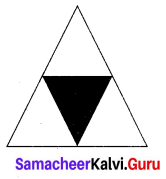Students can Download Maths Chapter 2 Percentage and Simple Interest Ex 2.2 Questions and Answers, Notes Pdf, Samacheer Kalvi 7th Maths Book Solutions Guide Pdf helps you to revise the complete Tamilnadu State Board New Syllabus and score more marks in your examinations.

## Tamilnadu Samacheer Kalvi 7th Maths Solutions Term 3 Chapter 2 Percentage and Simple Interest Ex 2.2

Question 1.
Write each of the following percentage as decimal.
(i) 21 %
(ii) 93.1 %
(iii) 151 %
(iv) 65 %
(v) 0.64 %
Solution:
(i) 21 %
= $$\frac { 21 }{ 100 }$$ = 0.21

(ii) 93.1 %
= $$\frac { 93.1 }{ 100 }$$ = 0.931

(iii) 151 %
= $$\frac { 151 }{ 100 }$$ = 1.51

(iv) 65 %
= $$\frac { 65 }{ 100 }$$ = 0.65

(v) 0.64 %
= $$\frac { 0.64 }{ 100 }$$ = 0.0064Question 2.
Convert each of the following decimal as percentage
(i) 0.282
(ii) 1.51
(iii) 1.09
(iv) 0.71
(v) 0.858
Solution:
(i) 0.282
= 0.282 × 100% = $$\frac { 282 }{ 1000 }$$ × 100 %
= 28.2 %

(ii) 1.51
= $$\frac { 151 }{ 100 }$$ × 100 %
= 151 %

(iii) 1.09
= $$\frac { 109 }{ 100 }$$ × 100 %
= 109 %

(iv) 0.71
= $$\frac { 71 }{ 100 }$$ × 100 %
= 71 %

(v) 0.858
= $$\frac { 858 }{ 1000 }$$ × 100 %
= 85.8 %

Question 3.
In an examination a student scored 75% of marks. Represent the given the percentage in decimal form?
Solution:
Student’s Score = 75% = $$\frac { 75 }{ 100 }$$ = 0.75

Question 4.
In a village 70.5% people are literate. Express it as a decimal.
Solution:
Percentage of literate people = 70.5%
= $$\frac { 70.5 }{ 100 }$$
= 0.705Question 5.
Scoring rate of a batsman is 86%. Write his strike rate as decimal.
Solution:
Scoring rate of the batsman = 86%
= $$\frac { 86 }{ 100 }$$
= 0.86

Question 6.
The height of a flag pole in school is 6.75m. Write it as percentage.
Solution:
Height of flag pole = 6.75m
= $$\frac { 675 }{ 100 }$$
= 6.75%

Question 7.
The weights of two chemical substances are 20.34 g and 18.78 g. Write the difference in percentage?
Solution:
Weight of substance 1 = 20.34g
Percentage of substance 1 = $$\frac { 2034 }{ 100 }$$ = 2034 %
Weight of substance 2 = 18.78g
Percentage of substance 2 = $$\frac { 1878 }{ 100 }$$ = 1878 %
Their difference = 2034 – 1878 = 156%Question 8.
Find the percentage of shaded region in the following figure.Solution:
Total region = 4 parts
Fraction of shaded region = $$\frac { 1 }{ 4 }$$
Percentage of shaded region = $$\frac { 1 }{ 4 }$$ × $$\frac { 100 }{ 100 }$$
= $$\frac { 1 }{ 4 }$$ × 100 %
= 25 %

Objective Type Questions

Question 1.
Decimal value of 142.5% is
(i) 1.425
(ii) 0.1425
(iii) 142.5
(iv) 14.25
Hint:
142.5 % = $$\frac { 1425 }{ 10 }$$ %
= $$\frac { 1425 }{ 10 }$$ × $$\frac { 1 }{ 100 }$$
= 1.425
(i) 1.425Question 2.
The percentage of 0.005 is
(i) 0.005 %
(ii) 5 %
(iii) 0.5 %
(iv) 0.05 %
Hint:
0.005 = $$\frac { 5 }{ 1000 }$$
= $$\frac { 5 }{ 1000 }$$ × $$\frac { 100 }{ 100 }$$
= 0.5 %
(iii) 0.5 %

Question 3.
The percentage of 4.7 is
(i) 0.47 %
(ii) 4.7 %
(iii) 47 %
(iv) 470 %
Hint:
4.7 = $$\frac { 47 }{ 10 }$$
= $$\frac { 47 }{ 10 }$$ × $$\frac { 100 }{ 100 }$$
= 470 %